Next: Corollary 1.2 Up: Proof that Algorithm Increases Previous: Theorem 1   Contents

### Corollary 1.1

Corollary 1.1: We seek to show that after applying A, both k' and l' are positive, under the following conditions:

Let the state vector for:

• one state have amplitude k
• each of the remaining (N - 1) states the amplitude is l
And let:
• k and l be real
• k be negative, and l be positive
•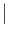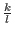<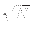• N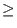9
Then, after applying A, both k' and l' are positive.

Proof:

First we will show that k' is positive:

• From theorem 1 we know k' =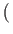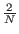- 1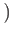k + 2l.

• By assumption k is negative. Since N > 2 by assumption,- 1is negative.

• By assumption l is positive. Since N > 2 by assumption, 2is positive.

• Thus the expression for k' is of the form negative*negative + positive*positive, which must be positive.

Next we will show that l' is positive:

• From theorem 1 we know l' =k +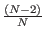l.

• By assumption<• For N9,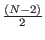>. Therefore when N9:>>, and:

l' =k +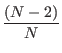l >k +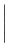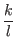l

• Because k is negative and l is positive by assumption,=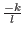.

• Therefore:

l' =k +l >k +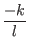l = (- 1)k

• It follows that l' is positive because k is positive and-1 > 0 for N3 (and by assumption N9).
[Grover96]Next: Corollary 1.2 Up: Proof that Algorithm Increases Previous: Theorem 1   Contents
Matthew Hayward - Quantum Computing and Grover's Algorithm GitHub Repository